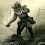## Saturday, February 2, 2013

### Chamberlain Mundlak Device and the Cluster Robust Hausman Test

* Unobserved variation can be divided in a useful manner.

* y_it = X_it*B + c_i + u_it

* c_i is fixed individual effect or random individual effect if c_i is uncorrelated with Xi (the time averages of X_it).

* In order for c_it to bias B is if there is some correlation between X_it and c_i.

* Therefore if we were to create a new variable which "controls" any time constant variation in X_it then the remaining v_i must be uncorrelated with X_it.

* Thus the Chamberlain Mundlak Device was born.

* Let's see it in action!

clear

set obs 100

gen id = _n

gen A1 = rnormal()
gen A2 = rnormal()

* Let's say we have 2 observations per individual
expand 2

gen x = rnormal()+A1
gen u = rnormal()*3

gen y = -5 +2*x + A1 + A2 + u

* Now in the above model there is both a portion of the unobserved variance correlated with the average x (A1) and a random portion uncorrelated with the average x (A2) the individual level.

* The fixed effect varies with the x variable while the random one does not.

* The standard approach in this case would be to use the Hausman test to differentiate between fixed effect and random effect models.

xtset id
* Let's first set id as the panel data identifier.

xtreg y x, fe
estimates store fe
* We store the estimates for use in the Hausman test

xtreg y x, re

hausman fe, sigmamore
* We strongly reject the null which we should expect so in classical econometric reasoning we choose to use the fixed effect estimator.

* An alternative method of estimating the fe estimator is by constructing the Chamberlain-Mundlak device.

* This device exploits the knowledge that the only portion of the time constant variation in X that can be correlated with u must be correlated only with the time average X for each individual.

bysort id: egen x_bar = mean(x)

reg y x x_bar

* Amazingly we can see that the new estimator is the same as the fe estimator above.

* Notice however the degrees of freedom.

* In the fe esimator we have used up half of our degrees of freedom.

* Yet our x estimate is the same size and our standard errors are very similar?

* If we double our sample size should not our standard errors decrease substantially?

* The answer is no. Why?

* I am going to run the fixed effect estimator manually.

reg y x i.id

* Look at our SSE or the R2.  In the fixed effect model the R2 is much larger.

* This is because in terms of the random effect (A2), the fixed effect model controls for both the portion of the unobserved individual level variance which is correlated with the average x for each student as well as that portion uncorrelated with the average x.

* The Chamberlain-Mundlak (CM) device however only controls for the portion of the variance correlated with the average Xs.  Thus there is much more unexplained variance which ends up reducing the power of our test which is approximately accounted for when we adjust the sample size.

* The CM can be additionally useful because it provides an alternative form of the Hausman test.

reg y x x_bar

* The significance of the generated regressor x_bar indicates the exogeneity of the unobserved individual effects.

* The test can be easily adjusted to be robust to cluster effects by specifying cluster in the regression.

reg y x x_bar, cluster(id)

1.2.